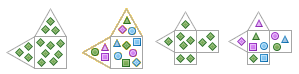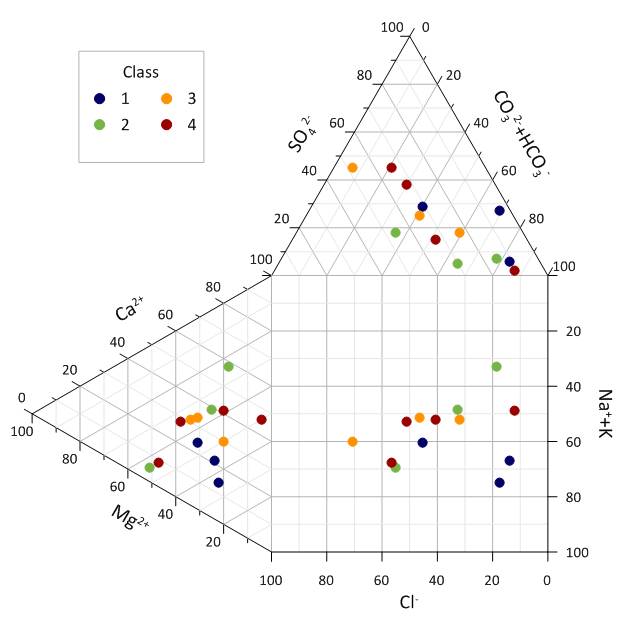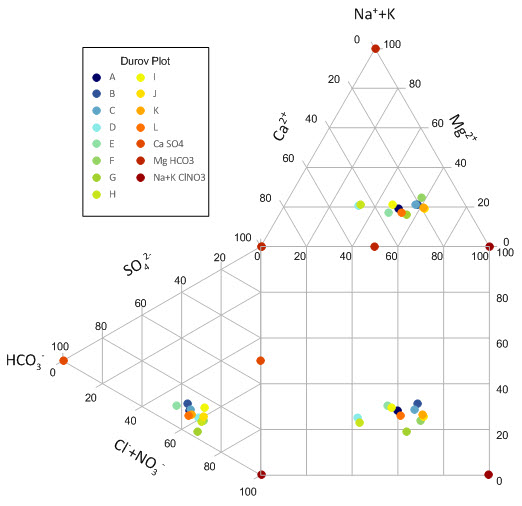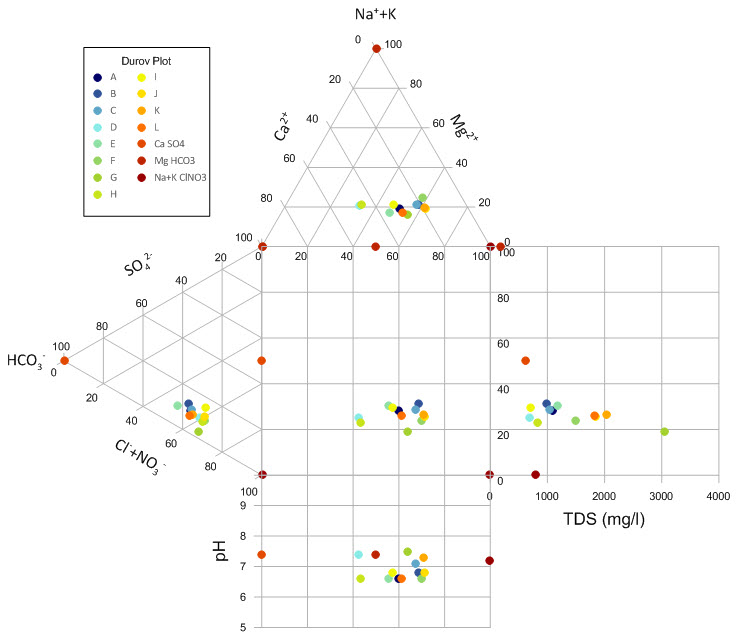Follow

# Can Grapher create a Durov diagram? If so, how?

Durov diagrams are a new plot type added to Grapher 14.  To create a Durov Plot in Grapher, complete the steps below.
1. Click the desired Home | New Graph | Ternary | Durov <plot type> command ().
2. In the Open Worksheet dialog, select your data file and click Open. The durov plot is created with the data columns assigned in the following order: cation X, cation Z, cation Y, anion X, anion Z, anion Y, pH (if applicable), TDS (if applicable),and class (if applicable).
3. If needed, adjust the data columns in the Plot tab of the Property Manager after clicking on the Cation or Anion Plot in the Object Manager.Grapher 13 and earlier versions cannot directly create a Durov diagram. However, the parts of a Durov diagram are created using graph types that Grapher does support. So, through a template graph and data file, Grapher can create this plot. A sample GRF and data file are attached.

The sample data file contains the recorded anion and cation concentrations in mg/l (or ppm) in columns B, C, D, E, I, J, K, and L. These columns and the ID in column A can be changed to any data desired. In addition, the values in the pH column can be entered by the user.

The Excel file has some calculations that are used for the ternary diagrams and the rectangular portions between the triangles. The input values are normalized in columns F, G, and H for the cations and in columns M, N, and O for the anions. The values are normalized again in columns P and Q for display in the rectangle between the triangles. In addition, the TDS is calculated by summing all of the mg/l columns. If the number of data points entered is greater than the existing rows, copy the equations for columns F, G, H, M, N, O, P and Q for all new data.

Once the data is input, open the GRF file in Grapher. The graph is automatically populated with the data.If you want to see the extended Durov plot, with the TDS and pH, check the box in the Object Manager next to Extended Durov and those additional fields will display.Updated October 12, 2018